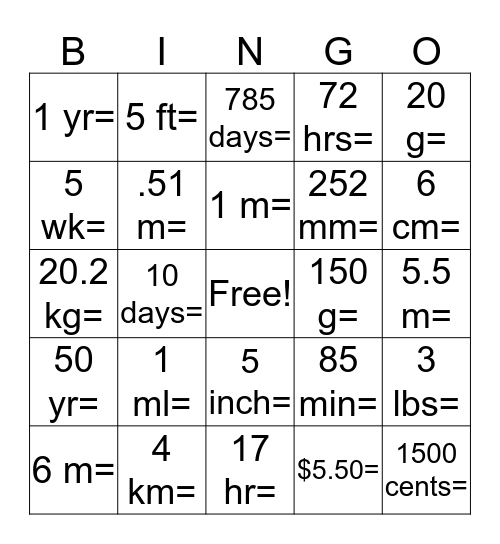# Unit Conversion BingoThis bingo card has a free space and 24 words: 1 ml=, 4 km=, 1 m=, 6 m=, 20 g=, 1 yr=, 5 wk=, 5 ft=, 150 g=, 5 inch=, 252 mm=, 20.2 kg=, 85 min=, 3 lbs=, .51 m=, \$5.50=, 5.5 m=, 785 days=, 6 cm=, 10 days=, 1500 cents=, 50 yr=, 72 hrs= and 17 hr=.

## Play Online